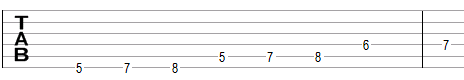# Harmonic minor scale

The harmonic minor scale is a heptatonic scale that can be built using the following steps between notes: 1, ½, 1, 1, ½, 1½, ½.

An example of the scale is A, B, C, D, E, F, G#. This example is shown below in traditional notation and in guitar tablature notation.The harmonic minor scale is the natural minor scale (A, B, C, D, E, F, G in this example), but with the raised seventh note (G# in this example).

The fifth mode of the harmonic minor scale uses the steps ½, 1½, ½, 1, ½, 1, 1 (E, F, G#, A, B, C, D in the example above) and is known as the Spanish gypsy scale because of its use in flamenco. Flamenco uses the Phrygian scale, but allows for alternating between a normal and a raised third on the Phrygian scale, and between a normal and a raised seventh on that scale. An example of the Phrygian is E, F, G, A, B, C, D and the raised third is the G# that results in the Spanish gypsy scale.

## Modes of the harmonic minor scale

The fifth mode of the harmonic minor scale is known as the Spanish gypsy scale, and has steps ½, 1½, ½, 1, ½, 1, 1 (E, F, G#, A, B, C, D in the example above).

## Three-note chords on the harmonic minor scale

The following are common triads built on the notes of the harmonic minor scale.

• On the root of the scale (on the tonic): minor chord (e.g., Am composed of A, C, E) or suspended chords (Asus4 = A, D, E and Asus2 = A, B, E).
• On the second note (on the supertonic): diminished chord (Bdim = B, D, F).
• On the third note (on the mediant): augmented chord (Caug = C, E, G#).
• On the fourth note (on the subdominant): minor chord (Dm = D, F, A), diminished chord (Ddim = D, F, G#), or suspended chord (Dsus2 = D, E, A).
• On the fifth note (on the dominant): major chord (E = E, G#, B), augmented chord (Eaug = E, G#, C), or suspended chord (Esus4 = E, A, B).
• On the sixth note (on the submediant): major chord (F = F, A, C), minor chord (Fm = F, G#, B), or diminished chord (Fdim = F, G#, B).
• On the seventh note (on the leading tone: augmented chord (G#aug = G#, C, E) or diminished chords (G#dim = G#, B, D).

## Four-note chords on the harmonic minor scale

The following are seventh chords built on the notes of the harmonic minor scale.

• On the first note: minor-major seventh chord (e.g. Ammaj7 composed of A, C, E, G#).
• On the second note: half-diminished seventh chord (minor seventh chord with a flat fifth, Bm7b5 = B, D, F, A) or a diminished seventh chord (minor seventh chord with a flat fifth and a diminished seventh, B*7 = B, D, F, G#).
• On the third note: augmented major seventh chord (Cmaj7#5 = C, E, G#, B).
• On the fourth note: minor seventh chord (Dmin7 = D, F, A, C) or half-diminished seventh chords (Dm7b5 = D, F, G#, C and D*7 = D, F, G#, B).
• On the fifth note: dominant seventh chord (E7 = E, G#, B, D) or augmented seventh chord (E7#5 = E, G#, C, D).
• On the sixth note: major seventh chord (Fmaj7 = F, A, C, E), half-diminished seventh chord (F*7 = F, G#, B, D), or minor-major seventh chord (Fmmaj7 = F, G#, C, E).
• On the seventh note: half-diminished seventh chord (G#*7 = G#, B, D, F).

## Intervals on the harmonic minor scale

The harmonic minor scale is composed of the following intervals.

• A major second, e.g., the interval between A and B is equal to two semitones.
• A minor third, e.g., the interval between A and C is equal to three semitones.
• A perfect fourth, e.g., the interval between A and D is equal to five semitones.
• A perfect fifth, e.g., the interval between A and E is equal to seven semitones.
• A minor sixth, e.g., the interval between A and F is equal to eight semitones.
• A major seventh, e.g., the interval between A and G# is equal to eleven semitones.

Scale, Scale (index)

### Filtered HTML

• Freelinking helps you easily create HTML links. Links take the form of [[indicator:target|Title]]. By default (no indicator): Click to view a local node.